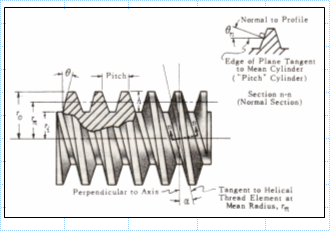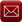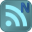You have downloaded this file 0 times in the last 24 hours, limit is 100.

## Power Screw.xls This location is for Registered Users Only.Perhaps you need to login or register.

Description:Purpose of calculation
: For an applied load on a screw thread calculate the axial thrust of a nut. Calculate bolt pretension from screw parameters (thread pitch, thread coefficient of friction, head coefficient of friction etc.).

Calculation Reference
Schaum's Outline - Machine Design

Submitted By:
Submitted On:
29 Jan 2008
File Size:
199.00Kb
483
File Version:
01
Rating:Total Votes:6
Willempi 10 years ago
Comment 1: Instead of using the long conversion of angle (in radians) to degrees, you can use the built-in conversion function =DEGREES(XX), where XX is the cell reference (radians), then you can add the cell formatting as per comment 2 below.
Comment 2: You can format the degrees as follows. Use Custom number formatting of the cell in which you display the degree equivalent of the angle (in radians) by typing 0.00_;"°" , which would display the unit "°" in the cell.
Willempi 10 years ago
Comment 1: Instead of using the long conversion of angle (in radians) to degrees, you can use the built-in conversion function =DEGREES(XX), where XX is the cell reference (radians), then you can add the cell formatting as per comment 2 below.
Comment 2: You can format the degrees as follows. Use Custom number formatting of the cell in which you display the degree equivalent of the angle (in radians) by typing 0.00_;"°" , which would display the unit "°" in the cell.
allenjray 11 years ago
thank-you
allenjray 11 years ago
thank-you
mstickma that's a useful link for many threadforms. The thread depth variable, t, in the calculation can be set to suit any particular threadform.
John,
Thanks for creating this spreadsheet. It has saved me the time of recreating it from a similar Mathcad file I was using.
I was wondering if you could elaborate on what the thread depth variable is in the sheet. I took it as 0.600 X P for metric fine threads. I found this at: [url]http://www.colinusher.info/Livesteam/mewdata.html#data[/url]
Is this correct?
There is a very useful tool on the Norbar website called the [url=http://www.norbar.com/torquetensioncalculator.php]Torque Tension Calculator[/url]. It provides a useful check and useful thread dimensions which can be inserted into the spreadsheet calculation.
ziggle 12 years ago
Thanks!
ziggle 12 years ago
Thanks!

We have 275 guests and 7 members onlineEmail (preferred method of contact)US +1 617 5008224EU +44 113 8152220